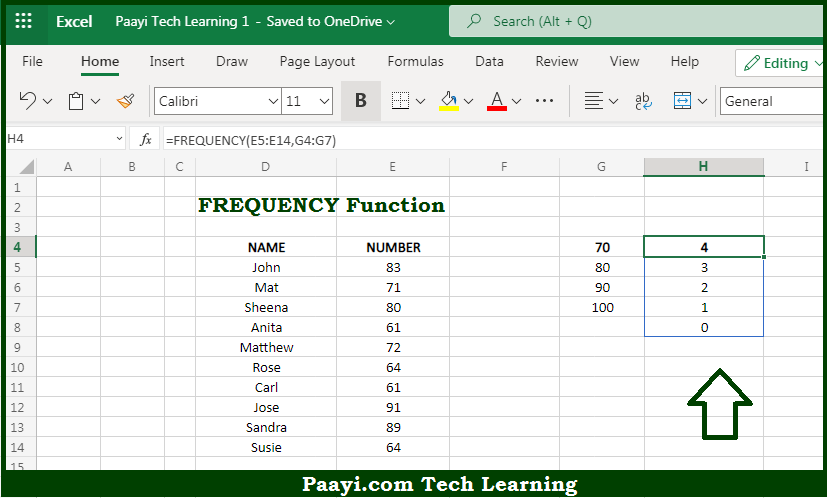# Learn How to Use Microsoft Excel FREQUENCY Function

Written by | 0 Comments | 581 Views

In this article, you will learn how to use the Microsoft Excel FREQUENCY function and its prime function in Microsoft Excel. You will also get to know the Microsoft Excel FREQUENCY function return value and syntax with the help of some examples.

Microsoft Excel FREQUENCY Function

The main purpose of the Microsoft Excel FREQUENCY function is to get the frequency of values in the given data set. That implies, with the help of the FREQUENCY function you can able to return a frequency distribution, which is a summary table that shows the frequency of each value in the given range. The FREQUENCY function returns multiple values and should be entered as an array formula with control-shift-enter, except in Excel 365. So, with the help of the FREQUENCY function, you can able to get the frequency of values in the given data set.

Return Value of FREQUENCY Function

The return value will be the vertical array of frequencies.

Syntax of FREQUENCY Function

=FREQUENCY(data-array, bins-array)

Where the arguments:

• data-array: This is an array of values for which you want to get frequencies.
• bins-array: This is the array of intervals or bins for grouping values.

## How to Use Microsoft Excel FREQUENCY Function?So we know that Microsoft Excel FREQUENCY function you can able to get the frequency of values in the given data set. That implies, with the help of the FREQUENCY function you can able to return a frequency distribution, which is a summary table that shows the frequency of each value in the given range. The FREQUENCY function returns multiple values and should be entered as an array formula with control-shift-enter, except in Excel 365. So, with the help of the FREQUENCY function, you can able to get the frequency of values in the given data set.